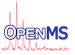OpenMS  2.8.0
PScore Struct Reference

Implementation of the PScore PSM scoring algorithm. More...

`#include <OpenMS/ANALYSIS/RNPXL/PScore.h>`

## Static Public Member Functions

static std::vector< SizecalculateIntensityRankInMZWindow (const std::vector< double > &mz, const std::vector< double > &intensities, double mz_window)
calculate local (windowed) peak ranks. More...

static std::vector< std::vector< Size > > calculateRankMap (const PeakMap &peak_map, double mz_window=100)
precalculated, windowed peak ranks for a whole experiment. More...

static std::map< Size, PeakSpectrumcalculatePeakLevelSpectra (const PeakSpectrum &spec, const std::vector< Size > &ranks, Size min_level=1, Size max_level=9)
Calculates spectra for peak level between min_level to max_level and stores them in the map A spectrum of peak level n retains the (n+1) top intensity peaks in a sliding mz_window centered at each peak. More...

static double computePScore (double fragment_mass_tolerance, bool fragment_mass_tolerance_unit_ppm, const std::map< Size, PeakSpectrum > &peak_level_spectra, const std::vector< PeakSpectrum > &theo_spectra, double mz_window=100.0)
Computes the PScore for a vector of theoretical spectra. More...

static double computePScore (double fragment_mass_tolerance, bool fragment_mass_tolerance_unit_ppm, const std::map< Size, PeakSpectrum > &peak_level_spectra, const PeakSpectrum &theo_spectrum, double mz_window=100.0)
Computes the PScore for a single theoretical spectrum. More...

static double massCorrectionTerm (double mass)

static double cleavageCorrectionTerm (Size cleavages, bool consecutive_cleavage)

static double modificationCorrectionTerm (Size modifications)

## Detailed Description

Implementation of the PScore PSM scoring algorithm.

## ◆ calculateIntensityRankInMZWindow()

 static std::vector calculateIntensityRankInMZWindow ( const std::vector< double > & mz, const std::vector< double > & intensities, double mz_window )
static

calculate local (windowed) peak ranks.

The peak rank is defined as the number of neighboring peaks in +/- (mz_window/2) that have higher intensity The result can be used to efficiently filter spectra for top 1..n peaks in mass windows

Note
ranks are zero based (highest intensity peak in window has rank 0)
Parameters
 mz m/z positions of the peaks intensities of the peaks mz_window window in Thomson centered at each peak

## ◆ calculatePeakLevelSpectra()

 static std::map calculatePeakLevelSpectra ( const PeakSpectrum & spec, const std::vector< Size > & ranks, Size min_level = `1`, Size max_level = `9` )
static

Calculates spectra for peak level between min_level to max_level and stores them in the map A spectrum of peak level n retains the (n+1) top intensity peaks in a sliding mz_window centered at each peak.

Note
levels are zero based (level 0 has only the top intensity peaks for each window, level 1 the top and second most intensive one)
min and max level are taken from the Andromeda publication but are similar to the AScore publication

## ◆ calculateRankMap()

 static std::vector > calculateRankMap ( const PeakMap & peak_map, double mz_window = `100` )
static

precalculated, windowed peak ranks for a whole experiment.

The peak rank is defined as the number of neighboring peaks in +/- (mz_window/2) that have higher intensity

1. Each spectrum is subdivided into windows of size `mz_window`.
2. For each window, peak ranks are assigned using calculateIntensityRankInMZWindow().
3. A rank map is returned
Note
ranks are zero based (top element has rank 0)
Parameters
 peak_map Fragment spectra used for rank calculation. Typically a peak map after removal of all MS1 spectra. mz_window window in Thomson centered at each peak

## ◆ cleavageCorrectionTerm()

 static double cleavageCorrectionTerm ( Size cleavages, bool consecutive_cleavage )
static

correction term for type of cleavage. For reference see the Andromeda source code.

Note
constants used in the correction term might be instrument dependent

## ◆ computePScore() [1/2]

 static double computePScore ( double fragment_mass_tolerance, bool fragment_mass_tolerance_unit_ppm, const std::map< Size, PeakSpectrum > & peak_level_spectra, const PeakSpectrum & theo_spectrum, double mz_window = `100.0` )
static

Computes the PScore for a single theoretical spectrum.

Parameters
 fragment_mass_tolerance mass tolerance for matching peaks fragment_mass_tolerance_unit_ppm whether Thomson or ppm is used peak_level_spectra spectra for different peak levels (=filtered by maximum rank). theo_spectrum Theoretical spectrum as obtained e.g. from TheoreticalSpectrumGenerator mz_window window in Thomson centered at each peak

## ◆ computePScore() [2/2]

 static double computePScore ( double fragment_mass_tolerance, bool fragment_mass_tolerance_unit_ppm, const std::map< Size, PeakSpectrum > & peak_level_spectra, const std::vector< PeakSpectrum > & theo_spectra, double mz_window = `100.0` )
static

Computes the PScore for a vector of theoretical spectra.

Similar to Andromeda, a vector of theoretical spectra can be provided that e.g. contain loss spectra or higher charge spectra depending on the sequence. The best score obtained by scoring all those theoretical spectra against the experimental ones is returned.

Parameters
 fragment_mass_tolerance mass tolerance for matching peaks fragment_mass_tolerance_unit_ppm whether Thomson or ppm is used peak_level_spectra spectra for different peak levels (=filtered by maximum rank). theo_spectra theoretical spectra as obtained e.g. from TheoreticalSpectrumGenerator mz_window window in Thomson centered at each peak

## ◆ massCorrectionTerm()

 static double massCorrectionTerm ( double mass )
static

additive correction terms used by Andromeda (pscore + massC + cleaveC + modC - 100). For reference see the Andromeda source code.

Note
constants used in the correction term might be instrument dependent

## ◆ modificationCorrectionTerm()

 static double modificationCorrectionTerm ( Size modifications )
static

correction term for modification. For reference see the Andromeda source code.

Note
constants used in the correction term might be instrument dependent